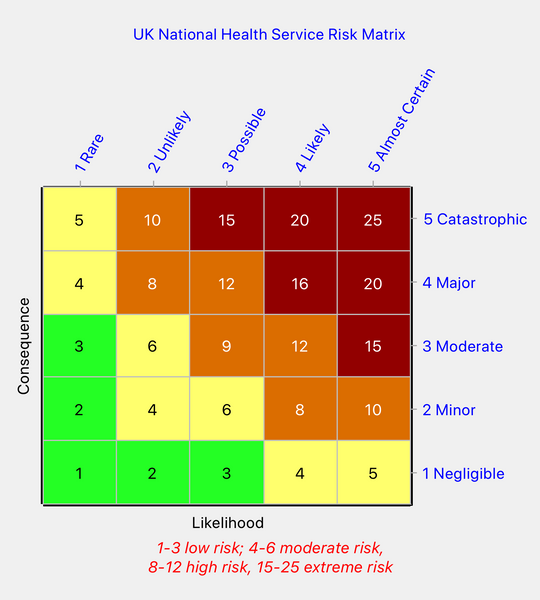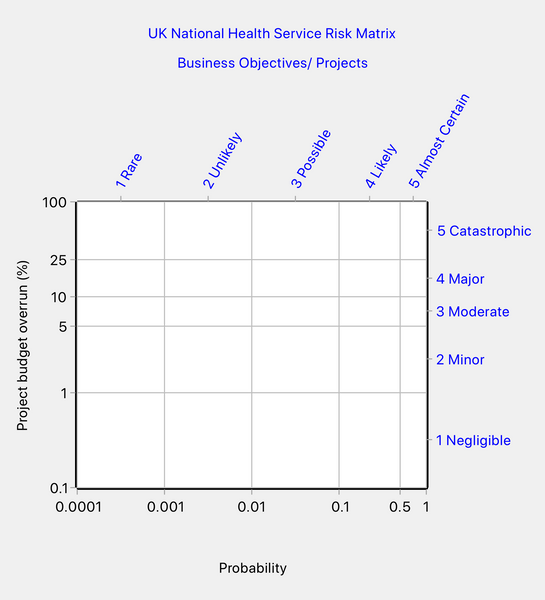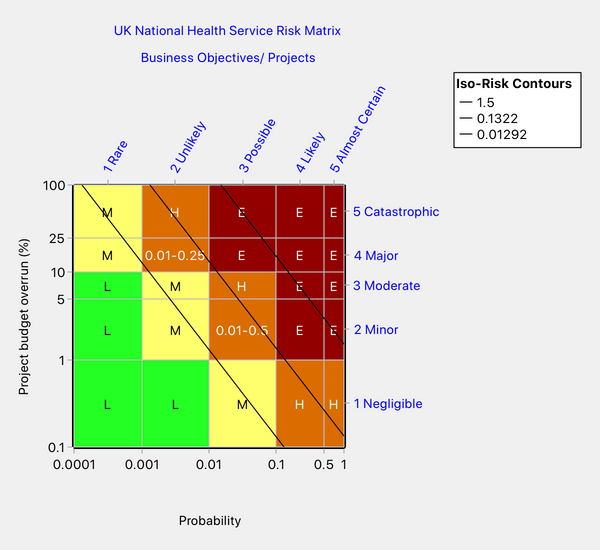# How Not to Design a Risk Matrix - National Health Service (UK) Risk Matrix

There are many ways in which to design a poor risk matrix, but one method is used so often that it deserves its own article. This unsound method involves the use of ordinal scales for likelihood and consequence and a pretense that the ordinal scales (which are essentially just labels) can express the magnitude of the likelihood and consequence variables.

A case in point is the risk matrix of the UK National Health Service (NHS). (Reference. A Risk Matrix for Risk Managers, NHS, National Patient Safety Agency, 2008. ) Introduced in 2008, the risk matrix continues to be used by health organizations across the United Kingdom:Figure 1: NHS risk matrix

Figure 1 shows the ordinal scales, which range from 1 to 5 on each axis. It also shows the score assigned by the NHS to each cell, which is the product of its column and row ordinal values, and the risk scores that define the four risk priority levels ("low," "moderate," "high" and "extreme" risk).

The NHS uses the same matrix for 10 types of consequence. It terms a consequence type a "domain." Two examples of domains are "Impact on the safety of patients, staff or public ..." and "Adverse publicity/ reputation." It also uses the same matrix in relation to three different likelihood scales: (1) broad linguistic descriptors of frequency (no numbers), (2) time-based descriptors ("daily", "weekly", "monthly", etc.), and (3) probability descriptors ("<0.1%", "0.1 to 1%", "1 to 10%", etc.).

It is not explained why the risk matrix coloring should be expected to be the same for 10 different consequence domains and three different likelihood scales. And nor is it explained why the thresholds between different risk priority levels should also be the same regardless of the consequence domain or frequency scale.

Rational risk matrix design depends on the use of ratio (quantitative) scales for likelihood and consequence. If we are to audit the NHS matrix, we need to identify the ratio scales that underlie it. Unfortunately, for most of the consequence domains, the descriptors are linguistic, not quantitative. There are a few exceptions, however, so all is not lost.

We will consider the consequence domain called "business objectives/projects." It appears that this domain is intended to be used in conjunction with the probability descriptors of likelihood. Although we can extract most of the axis tick mark values from the NHS documentation, the upper and lower bounds of the scales are undefined, so we are forced to make some reasonable assumptions. For example, the "Catastrophic" consequence is defined as a budget overrun greater than 25%, so we have assumed that that category could range from 25% to 100%. It is not widely appreciated that the bounds do matter and can affect the coloring of the top and bottom rows and the left and right columns. So, with reasonable assumptions, the scales for this case look something like this (plotted on log scales):Figure 2: NHS risk matrix - scales for business objectives/projects

To design a risk matrix, we also need to know the values of the iso-risk contours that separate the different risk priority levels. That information was not provided but we can make some assumptions and sensitivity studies. We quickly discover that any design we produce using the above scales is not going to be symmetrical, in contrast to the NHS matrix which is completely symmetrical.

We assume in this audit that risk should be calculated as the product of probability and consequence, as is conventional. We can be confident this is how the NHS views risk since we have seen that the NHS multiplies row and column numbers to obtain a surrogate for risk and so is definitely not using a more sophisticated definition.

One attempt to reproduce the NHS matrix looks as shown below in Figure 3. We used the Predominant Color algorithm of Quick Risk Matrix for this design. With that algorithm, each cell split by an iso-risk contour takes the color of the risk priority level that occupies the largest area of the cell.Figure 3: An attempt to reproduce the NHS risk matrix

We arrived at the matrix in Figure 3 by positioning the two lowest contours so that columns 1 and 2 match the NHS design and by positioning the highest contour so that row 5 matches the NHS design. But it is evident that we cannot match the entire matrix and no amount of playing with the contour values is going to produce a complete match. We also tried other coloring algorithms with no better result.

Thus, it appears that the standard NHS matrix is not consistent with this particular set of axis scales. That suggests that we might be able to find inconsistencies in the NHS matrix and indeed we can. Look at the two cells marked up in the following figure:Figure 4: Two cells in our redesign that the NHS risk matrix (Figure 1) rates inconsistently

Our design in Figure 4 identifies two cells in risk priority level "High" that the NHS matrix in Figure 1 colors inconsistently. Note that he cell in column 2, row 4, contains points corresponding to risks ranging from 0.01 to 0.25, while the cell in column 3, row 2, contains risks from 0.01 to 0.5. The NHS risk matrix (Figure 1) ranks the former cell as "High" risk and the latter as "Moderate" risk. Since the two cells have the same lower bound of 0.01 but the latter has a greater upper bound, then the latter cell cannot have a lower risk rating than the former, as it does in the NHS matrix.

There are other inconsistencies in the NHS matrix but we shall be content to just give this one example.

Now suppose we accept Figure 4 as the "correct" coloring (we put "correct" in quotes because there is more than one possible design). Figure 4 is internally consistent, unlike the NHS matrix. Now suppose we consider a different consequence domain, with an axis having different subdivisions and/or with different values for the iso-risk contours separating the risk priority levels. There is a very good chance that we will find that a different coloring pattern would be required.

One may change an axis by a scaling factor without changing the coloring pattern, provided the risk thresholds are scaled by the same factor. For example, it makes no difference to the risk matrix design whether we use dollars or pesetas for a money axis. The same is true for scaling factors generally. But once the relative widths of the axis subdivisions differ, then the required coloring pattern will likely differ. The safest approach is to use Quick Risk Matrix to verify the matrix design in relation to all the scales with which it is to be used.